Finding derivative of a function by chain rule

Chapter 5 Class 12 Continuity and Differentiability
Concept wise

Differentiation forms the basis of calculus, and we need its formulas to solve problems.

We have prepared a list of all the Formulas

## Basic Differentiation Formulas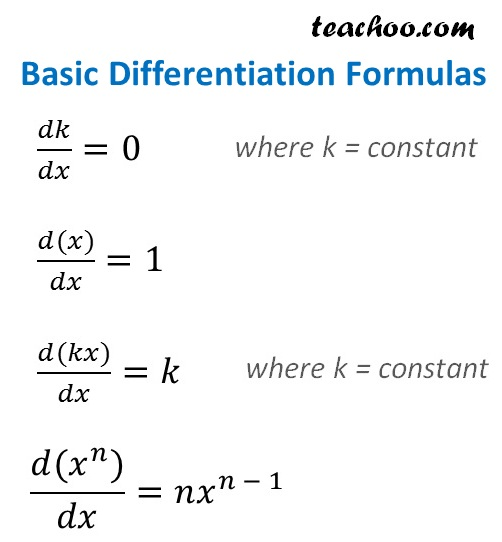## Differentiation of Log and Exponential Function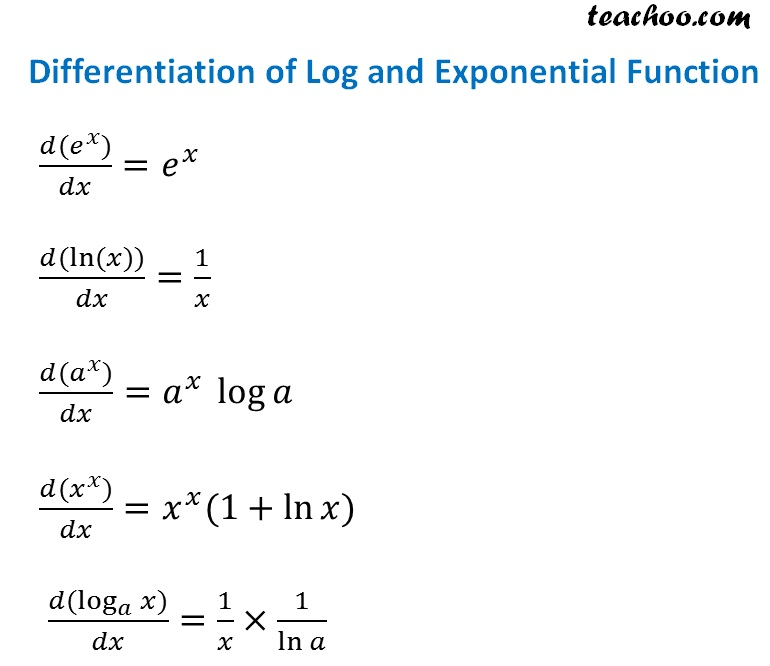## Differentiation of Trigonometry Functions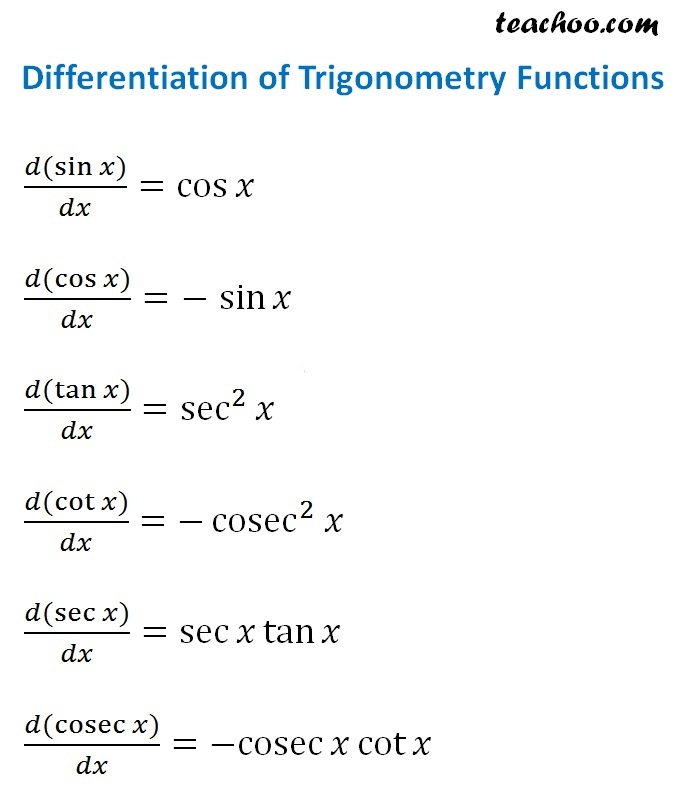## Differentiation of Inverse Trigonometry Functions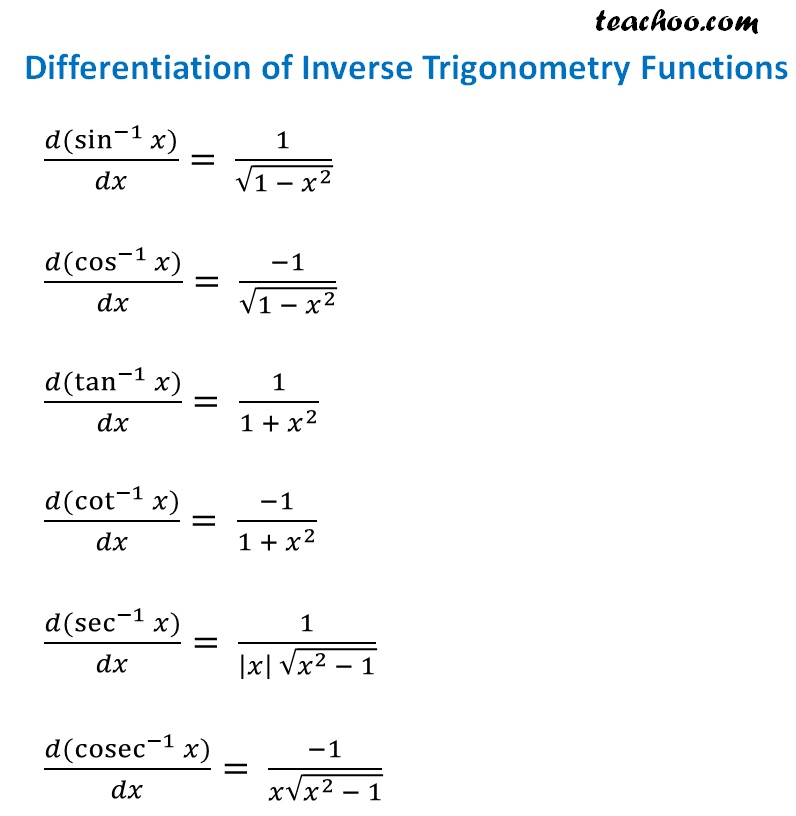## Differentiation Rules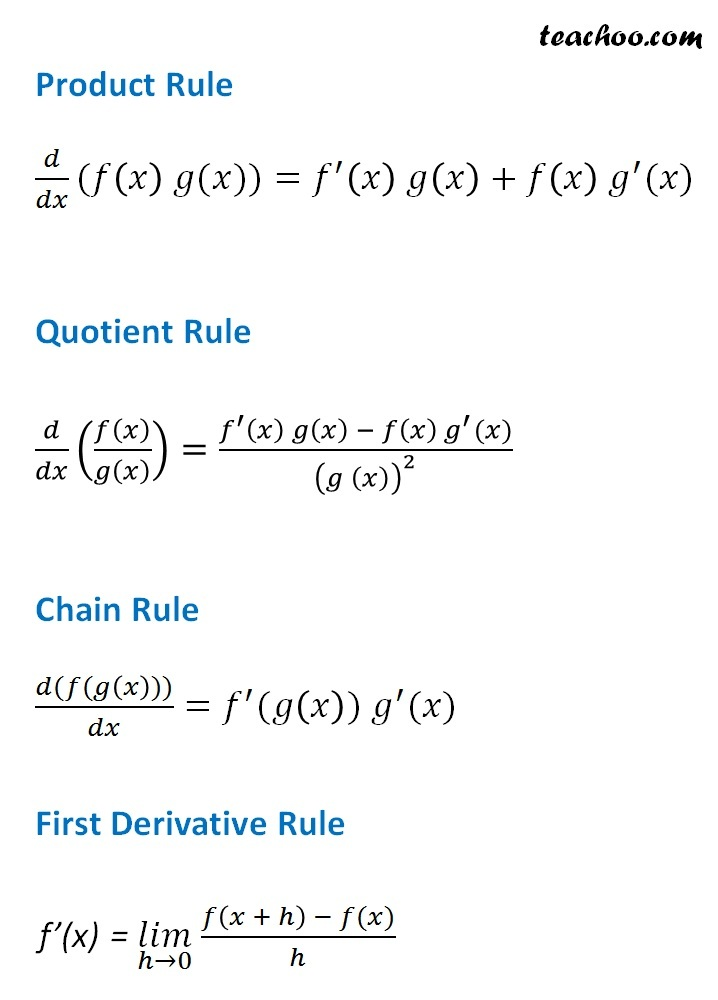Get live Maths 1-on-1 Classs - Class 6 to 12

### Transcript

𝑑𝑘/𝑑𝑥=0 (𝑑(𝑥))/𝑑𝑥=1 (𝑑(𝑘𝑥))/𝑑𝑥=𝑘 (𝑑(𝑥^𝑛))/𝑑𝑥=𝑛𝑥^(𝑛 − 1) (𝑑(𝑒^𝑥))/𝑑𝑥=𝑒^𝑥 (𝑑(ln⁡〖(𝑥)〗))/𝑑𝑥=1/𝑥 (𝑑(𝑎^𝑥))/𝑑𝑥=𝑎^𝑥 〖 log〗⁡𝑎 (𝑑(𝑥^𝑥))/𝑑𝑥=𝑥^𝑥 (1+ln⁡𝑥) (𝑑(log_𝑎⁡𝑥))/𝑑𝑥=1/𝑥×1/ln⁡𝑎 (𝑑(sin⁡𝑥))/𝑑𝑥=cos⁡𝑥 (𝑑(cos⁡𝑥))/𝑑𝑥=sin⁡𝑥 (𝑑(tan⁡𝑥))/𝑑𝑥=sec^2⁡𝑥 (𝑑(cot⁡𝑥))/𝑑𝑥=−cosec^2⁡𝑥 " " (𝑑(sec⁡𝑥))/𝑑𝑥=sec⁡𝑥 tan⁡𝑥 (𝑑(cosec⁡𝑥))/𝑑𝑥=〖−cosec〗⁡𝑥 cot⁡𝑥 (𝑑(sin^(−1)⁡𝑥))/𝑑𝑥= 1/√(1 − 𝑥^2 ) (𝑑(cos^(−1)⁡𝑥))/𝑑𝑥= (−1)/√(1 − 𝑥^2 ) (𝑑(tan^(−1)⁡𝑥))/𝑑𝑥= 1/(1 + 𝑥^2 ) (𝑑(cot^(−1)⁡𝑥))/𝑑𝑥= (−1)/(1 +〖 𝑥〗^2 ) (𝑑(sec^(−1)⁡𝑥))/𝑑𝑥= 1/(|𝑥| √(𝑥^2 − 1)) (𝑑(cosec^(−1)⁡𝑥))/𝑑𝑥= (−1)/(𝑥√(𝑥^2 − 1)) Product Rule 𝑑/𝑑𝑥(𝑓(𝑥) 𝑔(𝑥))=𝑓^′ (𝑥) 𝑔(𝑥)+𝑓(𝑥) 𝑔^′ (𝑥) Quotient Rule 𝑑/𝑑𝑥 (𝑓(𝑥)/𝑔(𝑥) )=(𝑓^′ (𝑥) 𝑔(𝑥) − 𝑓(𝑥) 𝑔^′ (𝑥))/(𝑔 (𝑥))^2 Chain Rule (𝑑(𝑓(𝑔(𝑥))))/𝑑𝑥=𝑓^′ (𝑔(𝑥)) 𝑔^′ (𝑥) First Derivative Rule f’(x) = (𝑙𝑖𝑚)┬(ℎ→0) 𝑓⁡〖(𝑥 + ℎ) − 𝑓(𝑥)〗/ℎ